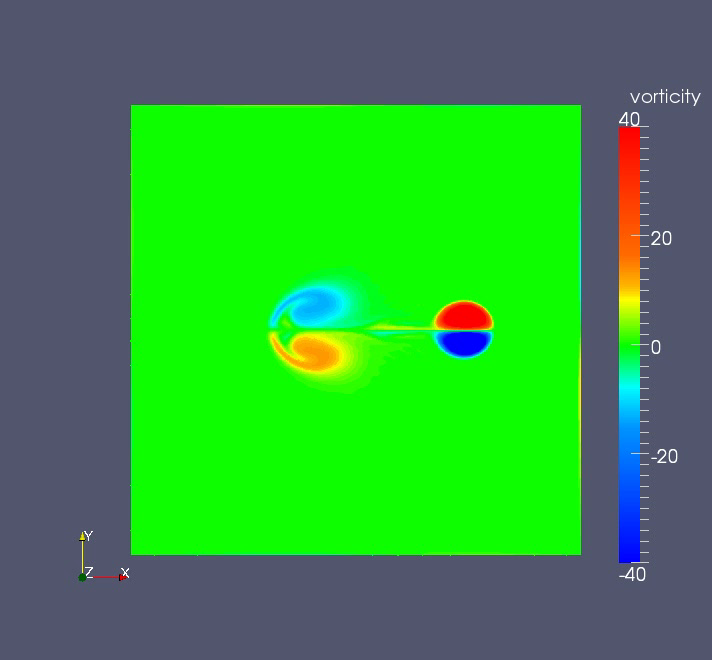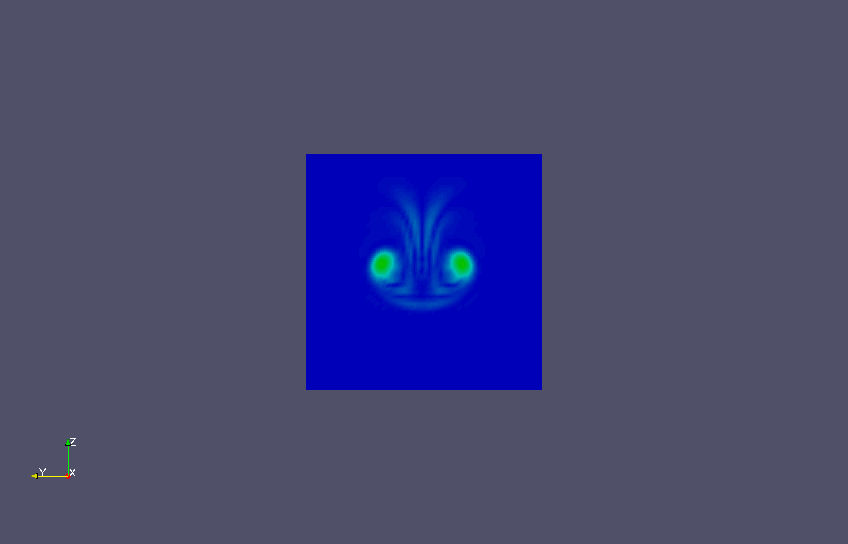During my Ph.D thesis, I developed wavelet methods for solving incompressible Navier-Stokes equations with physical boundary conditions. Below are examples of simulations obtained with my wavelet numerical code. For details see my thesis dissertation [tel-00544373] pdf.

### Lid driven cavity flow

The lid driven cavity flow is a benchmark problem widly used in the work of literature. We used this benchmark to evaluate the performance of our method. The divergence-free scaling function coefficients (left) correspond to a flow with Re=5000 simulated at j=8 space resolution. The vorticity (right) corresponds to a flow with Re=50000 simulated at j=9 space resolution. The vertical velocity profile is v=(-16x^2(1-x)^2,0).VideoVideo

### Vortex dipole rebound from a wall

This test allows us to evaluate the effect homogeneous Dirichlet boundary condition. The space resolution corresponds to j=9 and the used kinematic viscosity is nu=1/5000.Video

### 3D vortex tube reconnection

In this simulation we used periodic box, with 64^3 points. The first simulation (left) shows the isocontours of the vorticity and the second (right) shows the vorticity in the reconnection plan.VideoVideo